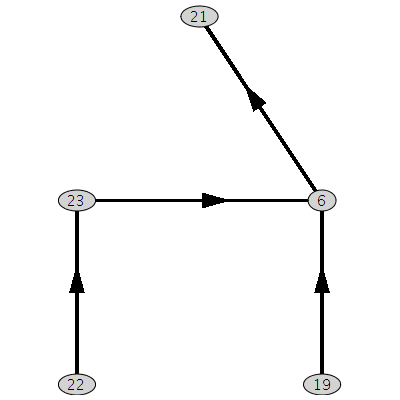# Network plot, Hasse diagram. browsing

• I have been using this to create a plot of a network:

$graph=plotmaple("plottools[rotate](GraphTheory[DrawNetwork](GraphTheory[Digraph]($cove),stylesheet=[arrowsize = 18]),Pi)");

The reason I rotate is because I was initially interested in plotting a Hasse diagram. However, it seems the DrawNetwork function does not really draw an acyclic graph by levels: an acyclic graph can be associated with a poset and as a consequence can be displayed by levels like in a Hasse Diagram, where the vertices with no ingoing arcs are displayed at the bottom level, then once these vertices are removed the remaining vertices with in-degree 0 are placed at the next level, and so on until all the vertices have ben placed. I would be grateful if there was a solution for this.

However the other issue is that I don't seeem to be able to reduce the thickness of the lines drawn. I tried the option thicness = 0 as part of the stylesheet list with no avail. The image is as follows:If I could create a proper Hasse diagram this would look like this:

• @Pierre

This would look like this: